Test: Hall Effect

# Test: Hall Effect

Test Description

## 10 Questions MCQ Test Electronic Devices | Test: Hall Effect

Test: Hall Effect for Electronics and Communication Engineering (ECE) 2023 is part of Electronic Devices preparation. The Test: Hall Effect questions and answers have been prepared according to the Electronics and Communication Engineering (ECE) exam syllabus.The Test: Hall Effect MCQs are made for Electronics and Communication Engineering (ECE) 2023 Exam. Find important definitions, questions, notes, meanings, examples, exercises, MCQs and online tests for Test: Hall Effect below.
Solutions of Test: Hall Effect questions in English are available as part of our Electronic Devices for Electronics and Communication Engineering (ECE) & Test: Hall Effect solutions in Hindi for Electronic Devices course. Download more important topics, notes, lectures and mock test series for Electronics and Communication Engineering (ECE) Exam by signing up for free. Attempt Test: Hall Effect | 10 questions in 30 minutes | Mock test for Electronics and Communication Engineering (ECE) preparation | Free important questions MCQ to study Electronic Devices for Electronics and Communication Engineering (ECE) Exam | Download free PDF with solutions
 1 Crore+ students have signed up on EduRev. Have you?
Test: Hall Effect - Question 1

### For which one of the following materials, is the Hall coefficient closest to zero?

Detailed Solution for Test: Hall Effect - Question 1

Hall coefficient is defined as: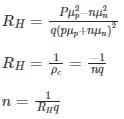ρc = charge density
σn = nμnq and σp = Pμpq
Hall coefficient depends on the hole and electron concentration, and also on the mobility of carriers.
In a metal, the gap between the conduction band and the valence band is very low.
The concentration of ions (n) is very high in metals.
So, the Hall coefficient will be zero almost for the metal as the Hall coefficient is inversely proportional to the concentration (n).

Test: Hall Effect - Question 2

### In Hall effect, a difference voltage is produced _______ to electric current in the conductor, and to an applied _____ field perpendicular to the current.

Detailed Solution for Test: Hall Effect - Question 2
• Hall effect is the production of a voltage difference across an electrical conductor that is transverse to an electric current and to an applied magnetic field perpendicular to the current
• When current flows in a semiconductor and magnetic field are applied perpendicularly, then the voltage is produced across the two side plates of the semiconductor bar, this is known as the Hall effect
• The Voltage produced is known as Hall voltage

The following diagram resembles an experimental setup for the observation of the Hall effect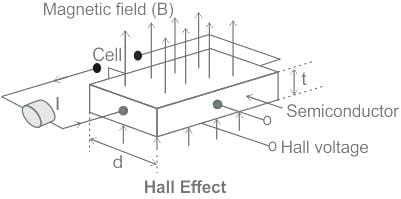Here we could observe that

• Hall voltage VH is developed transverse to the current in the conductor
• The hall voltage is also developed perpendicular to the applied magnetic field

Hall effect finds major applications in

• Hall sensors
• Used for proximity and displacement measurements
• Used in RPM,speed measurements
Test: Hall Effect - Question 3

### Hall effect is useful for the measurement of a semiconductor’s

Detailed Solution for Test: Hall Effect - Question 3

Hall Effect is basically the production of voltage (known as hall voltage) across conductor transverse to an electric current and to an applied magnetic field perpendicular to the current.
Application of Hall Effect:

• To determine the type of semiconductor
• To calculate the carrier concentration
• To determine mobility (hall mobility)
• To Measure magnetic flux density

From Hall Effect we have: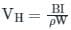Where the hall coefficient is given as: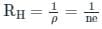Hall Effect can be used to measure the carrier concentration and type of semiconductor.

Test: Hall Effect - Question 4

Calculate the hall voltage when the magnetic field is 8 A/m, current is 4 A, width is 5 m and the concentration of carrier is 100000.

Detailed Solution for Test: Hall Effect - Question 4

The hall voltage VA is given by: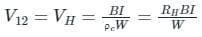ρc: Charge density = ne
RH: Hall coefficient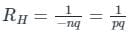W is the side across which the magnetic field is applied.
n is the carrier concentration
e is the electron charge
Calculation:
W = Thickness = 5 m, I = 4 A, B =  8 A/m, n = 100000
ρc = ne
ρc = 100000 × 1.6 × 10-19
ρc = 1.6 × 10-14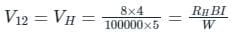Test: Hall Effect - Question 5

For an n-type Ge specimen, width = 4 mm, length = 1 mm, current (along the length of specimen) = 1 mA, magnetic field (perpendicular to the current flow direction) = 0.1 Wb/m2 and Hall voltage magnitude = 0.005 V. Calculate the majority carriers density.

Detailed Solution for Test: Hall Effect - Question 5

Concept:
The hall voltage VA is given by: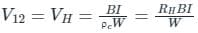ρc: Charge density
RH: Hall coefficient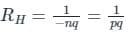W is the side across which the magnetic field is applied.
Calculation:
W = Thickness = 4 mm, I = 1 mA, VH = 0.005 V, B = 0.1 Wb/m2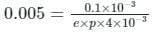Since hall voltage is positive, the majority charge carriers will be holes, with the concentration calculated as: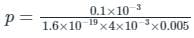p ≈ 3 × 1019 m-3

Test: Hall Effect - Question 6

For a particular material, the Hall coefficient is found to be zero. The material is

Detailed Solution for Test: Hall Effect - Question 6

Hall coefficient is defined as: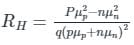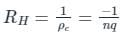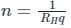We can find the electron concentration as: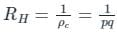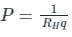ρc = charge density
σn = nμnq and σp = pμpq

• Hall coefficient depends on the hole and electron concentration, and also on the mobility of carriers.
• In an insulator, the gap between the conduction band and the valence band is very high.
• Conductivity will be almost zero in the insulator
• As conductivity zero in insulator then mobility also almost zero.
• So, the hall coefficient will be zero almost for the insulator.
Test: Hall Effect - Question 7

A hall’s effect pick-up can be used for measuring ________.

Detailed Solution for Test: Hall Effect - Question 7

Hall Effect Transducers:

• when a conductor is kept perpendicular to the magnetic field and a direct current is passed through it, It results in an electric field perpendicular to the directions of both the magnetic field and current with a magnitude proportional to the product of the magnetic field strength and current. The voltage so developed is very small and it is difficult to detect it. But in semiconductors such as germanium, this voltage is enough for measurement with a sensitive moving coil instrument. This phenomenon is called the Hall effect.
• Commercial Hall effect transducers are made from germanium or other semiconductor materials.
• Hall effect transducers find applications in instruments that measure magnetic field with small flux densities.
• Hall effect pick up can be used for measurement of current by magnetic field produced due to flow of current.
• Hall effect element may be used for measuring a linear displacement or location of the structural elements in cases where it is possible to change the magnetic field strength by variation in geometry of the magnetic structure.
• The main advantage of hall effect transducers is that they are non-contact device with small size and high resolution.
• The main drawback of hall effect transducers is highly sensitive to temperature variation and variation of Hall coefficient from plate to plate, thereby requiring individual calibration in each case.
Test: Hall Effect - Question 8

Which of the following quantities cannot be measured/determined using Hall Effect?

Detailed Solution for Test: Hall Effect - Question 8

Hall Effect: It states that if a “specimen (meta or semiconductor) carrying the current I is placed in traverse magnetic field B, then Electric field Intensity E is induced in a direction perpendicular to both I and B”.
Hall Voltage is given by: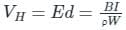The hall coefficient is given as: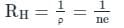Where,
ρ = charge density = σ / μ
n = charge concentration
σ = conductivity
μ = mobility constant
Hence Hall coefficient becomes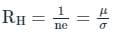From Hall Experiment, we can determine the following properties:

• Whether the specimen is a metal or semiconductor. In the case of semiconductors, we can find whether it is intrinsic, N-type, or P-type.
• The carrier concentration in the sample.
• Mobility of charge carriers.
• Used in designing Hall effect transducers.
• Hall voltage is induced in between the bottom and upper surfaces of the specimen.
• If the polarity of hall voltage is positive for the bottom surface, the given specimen is P-type.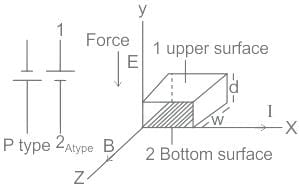Note: Diffusion constant is found from electron mobility using Einstein's relationship.

Test: Hall Effect - Question 9

Measurement of Hall coefficient enables the determination of:

Detailed Solution for Test: Hall Effect - Question 9

Hall Effect: It states that if a “specimen (meta or semiconductor) carrying the current I is placed in traverse magnetic field B, then Electric field Intensity E is induced in a direction perpendicular to both I and B”.

From Hall Experiment, we can determine the following properties:

• Whether the specimen is a metal or semiconductor. In the case of semiconductors, we can found whether it is intrinsic, N-type, or P-type.
• The carrier concentration in the sample.
• Mobility of charge carriers.
• Magnetic field intensity.
• Used in designing of Hall effect transducers.
• Hall voltage is induced in between the bottom and upper surfaces of the specimen.
• If the polarity of hall voltage is positive for the bottom surface, the given specimen is P-type.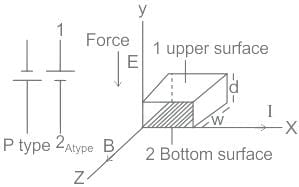From the above properties, we can say that, Option (b) is correct.

Test: Hall Effect - Question 10

The Hall Effect may be used to
1. Determine whether the semiconductor is p-type or n - type.
2. Determine the carrier concentration.
3. Calculate the mobilty.
Which of the above statements are correct?

Detailed Solution for Test: Hall Effect - Question 10

Hall Effect:
It states that if a specimen (metal or semiconductor) carrying a current (I) is placed in a transverse magnetic field (B), an electric field is induced in the direction perpendicular to both I and B.
Hall Voltage is given by: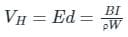The hall coefficient is given as: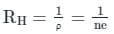Where,
ρ = charge density = σ / μ
n = charge concentration
σ = conductivity
μ = mobility constant
Hence Hall coefficient becomes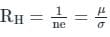• The Hall effect provides information on the sign, concentration, and mobility of charge carriers in the normal state.
• A positive sign for the Hall coefficient indicates that the majority carriers are holes and semiconductor is P-type.
• A negative sign for the Hall coefficient indicates that the majority carriers are electrons and semiconductor is N-type.

Applications of Hall-effect:
Hall effect can be used to find:
1. Carrier concentration
2. Type of semiconductor
3. Conductivity
4. Mobility
It cannot be used to find a magnetic field.

Common Confusion Point:
Looking at the formula one can think that the magnetic field can be calculated but in HALL Experiment, perpendicular MAGNETIC field and electric field are applied on the material and other parameters are measured.

## Electronic Devices

21 docs|20 tests
 Use Code STAYHOME200 and get INR 200 additional OFF Use Coupon Code
Information about Test: Hall Effect Page
In this test you can find the Exam questions for Test: Hall Effect solved & explained in the simplest way possible. Besides giving Questions and answers for Test: Hall Effect, EduRev gives you an ample number of Online tests for practice

21 docs|20 tests